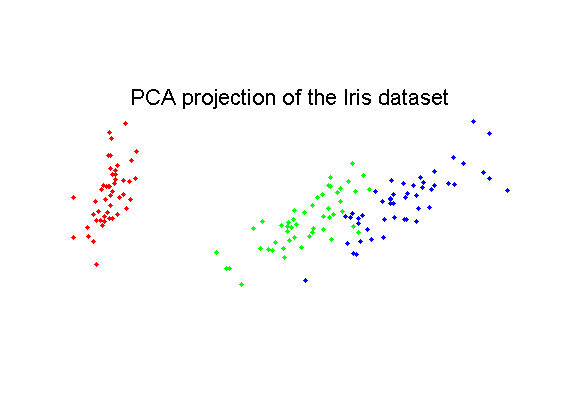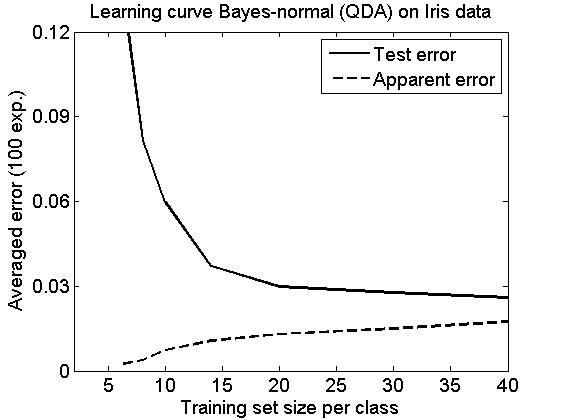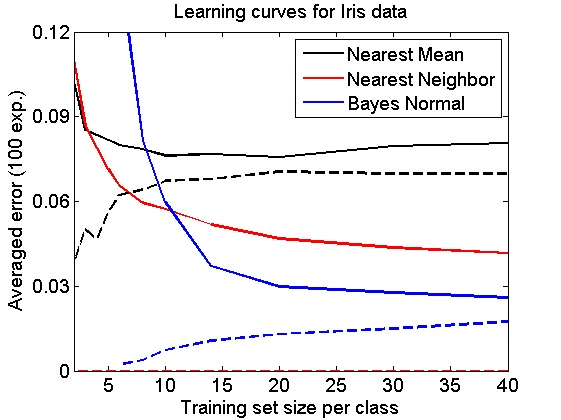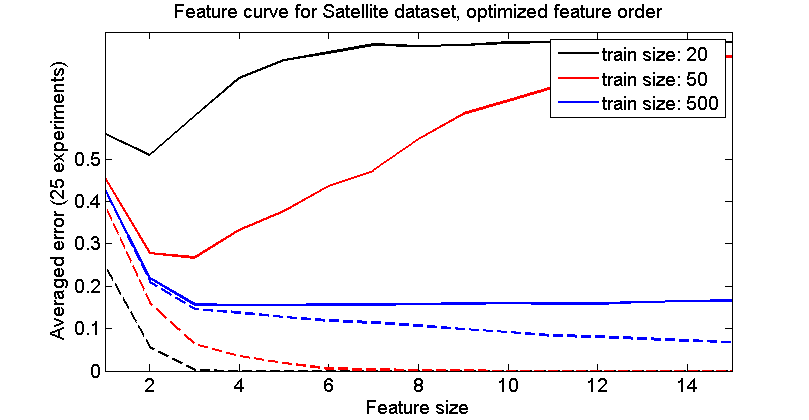# apperror

Examples of the behavior of the apparent error for increasing training set size, dimensionality and complexity.

PRTools and PRDataSets should be in the path

Download the m-file from here. See http://37steps.com/prtools for more.

## Show dataset

```delfigs
randreset(1)
a = iris;
a = setprior(a,0);
scattern(a*pcam(a,2));
title('PCA projection of the Iris dataset')
```## Show learning curve of qdc with apparent error

```figure
prwarning off;
e = cleval(a,qdc,[6 8 10 14  20 30 40],100);
plote(e,'nolegend')
legend('Test error','Apparent error')
title('Learning curve Bayes-normal (QDA) on Iris data')
ylabel('Averaged error (100 exp.)');
fontsize(14)
axis([2.0000 40.0000 0 0.1200])
```## Show learning curves of nmc and 1-nn

```e2 = cleval(a,nmc,[2 3 4 5 6 8 10 14  20 30 40],100);
e3 = cleval(a,knnc([],1),[2 3 4 5 6 8 10 14  20 30 40],100);
figure;
plote({e2,e3,e},'nolegend','noapperror')
title('Learning curves for Iris data')
ylabel('Averaged error (100 exp.)');
legend('Nearest Mean','Nearest Neighbor','Bayes Normal')
hold on;
plot([2 3 4 5 6 8 10 14 20 30 40],e2.apperror,'k--')
plot([2 3 4 5 6 8 10 14 20 30 40],e3.apperror,'r--');
plot([6 8 10 14 20 30 40],e.apperror,'b--');
linewidth(1.5)
fontsize(14)
axis([2.0000 40.0000 0 0.1200])
```Note that the apparent errors (dashed lines) are expected to increase with the size of the training set (more difficult for the classifier to classify all training objects correctly), but will decrease with the classifier complexity (more easy to classify the given training objects correctly).

## Show feature curves of satellite dataset

```figure;
a = satellite;
a = setprior(a,getprior(a));
w = featself(a,'maha-s');
trainsize = [20 50 500];
iter = 25;

x = a*w;
e4 = cell(1,numel(trainsize));
% prwaitbar calls are just used for reporting progress and may be skipped
[n,s,count] = prwaitbarinit('Processing %i curves:',2*numel(trainsize));
for j=1:numel(trainsize)
e4{j} = clevalf(x,remclass(2)*qdc([],[],1e-6),[1:15],trainsize(j),iter,[],testd);
count = prwaitbarnext(n,s,count);
end
h = plote(e4,'nolegend');
legend(h,'train size: 20','train size: 50','train size: 500')
title('Feature curve for Satellite dataset, optimized feature order')
fontsize(14)
set(gcf,'position',[ 680 558 808 420]);
```The apparent errors (dashed lines) decrease with growing classifier complexity and thereby also with growing dimensionality. For small training sets they decrease faster, as classifiers are then faster overtrained.